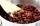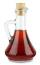# Mixtures and solutions + weighted average - math problems

#### Number of problems found: 14

• ThereThere is 5 l of water with a temperature of 20°C in the bucket. We add 3 liters of hot water. The resulting temperature will be 30°C. What was the temperature of the hot water?
• PharmacistThe pharmacist had a 12 l vessel herbal lotion, which contained a 30% active ingredient. Her assistant poured 18 liters of herbal lotion containing 45% of the active ingredient into a container. What percentage of herbal lotion was produced?
• Water mixingWe have 520 ml of hot water and 640 ml of water at 48°C. What is the temperature of approximately hot water when the resulting mixture has a temperature of 65°C?
• Mixture of nutsThe mixture of nuts should be prepared from almonds, peanuts and cashew nuts ratio 1: 2: 3 (respectively). The price of almonds is 150 CZK/kg, the price of peanuts is 140 CZK/kg and the price of cashew nuts is 180 CZK/kg. The price of the mixture is deter
• PeroxideHow many ml should we pour 30% of peroxide (H2O2) into 100ml H2O to give a 20% solution?
• CuSO4 mixtureHow many grams of solid CuSO4 we have to add to 450g of 15% CuSO4 solution to produce a 25% solution?
• Mixture 2How many liters of water must be added to 7 liters of a 20% solution to obtain a 10% solution?
• Dried fruitThe manufacturer produces a mixture of dried fruit. He purchased: 10kg pineapple for 200 Kc/kg 2kg papaya for 180 kc/kg 1kg of banana for 400 Kc/kg How many kgs of raisin for 80 Kc/kg must be put into the mix by the manufacturer so that the production pri
• PharmacyThe pharmacy adds to 3 liters of 95-percent alcohol 5 liters of 38.04 percent alcohol. How much percent alcohol the pharmacy got?
• Hydrochloric acidDetermine the concentration of which must have a solution of hydrochloric acid that mixing 10 l of the solution with 8 liters of 26% solution to get the solution with a concentration of 50%?
• VinegarWhat percentage vinegar we get if we mix 1 if dm³ eight percent vinegar with 1.5 dm³ six percent vinegar?
• Alcohol 2Two types of alcohol one 63% and second 75% give 20 liters of 69% alcohol. How many liters of each type are in the mixture?
• Vinegar 2How many percentages of vinegar solution will we get if we mix 3.5 liters of 5.8% and 5 liters of 7.6% vinegar?
• CoffeeIn-stock are three kinds of branded coffee prices: I. Kind. .. .. .205 Kc/kg II. Kind. .. .. .274 Kc/kg III. Kind. .. .. 168 Kc/kg Mixing these three species in the ratio 8:5:6 create a mixture. What will be the price of 100 grams of this mixture?

We apologize, but in this category are not a lot of examples.
Do you have an exciting math question or word problem that you can't solve? Ask a question or post a math problem, and we can try to solve it.

We will send a solution to your e-mail address. Solved examples are also published here. Please enter the e-mail correctly and check whether you don't have a full mailbox.

Mixtures and solutions - math problems. Weighted average - math problems.